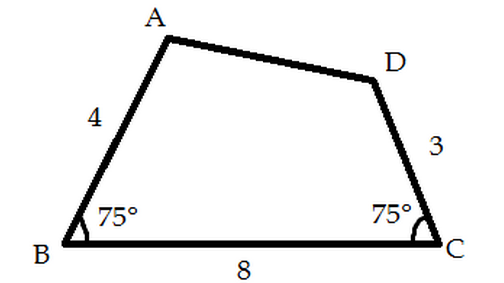# Can I make more assumptions?

Geometry Level 3Given that quadrilateral $ABCD$ with side length $AB=4, BC=8,CD=3$ and angles $B=C=75^\circ$, find the area of quadrilateral $ABCD$. Give your answer in unit squared.

Note: Figure not drawn up to scale.

×